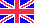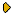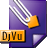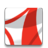Журнал Экспериментальной и Теоретической Физики
 НАЧАЛО | ПОИСК | ДЛЯ АВТОРОВ | ПОМОЩЬОбщая информация о журналеЗолотые страницыАдреса редакцииСодержание журналаСообщения редакцииПравила для авторовЗагрузить статьюПроверить статус статьиЖЭТФ, Том 141, Вып. 2, стр. 355 (Февраль 2012)
(Английский перевод - JETP, Vol. 114, No 2, p. 314, February 2012 доступен on-line на www.springer.com )

THERMODYNAMICS OF AN INTERACTING FERMI SYSTEM IN THE STATIC FLUCTUATION APPROXIMATION

Поступила в редакцию: 19 Апреля 2011DJVU (132K)PDF (482.2K)

We suggest a new method of calculation of the equilibrium correlation functions of an arbitrary order for the interacting Fermi-gas model in the framework of the static fluctuation approximation method. This method based only on a single and controllable approximation allows obtaining the so-called far-distance equations. These equations connecting the quantum states of a Fermi particle with variables of the local field operator contain all necessary information related to the calculation of the desired correlation functions and basic thermodynamic parameters of the many-body system. The basic expressions for the mean energy and heat capacity for the electron gas at low temperatures in the high-density limit were obtained. All expressions are given in the units of rs, where rs determines the ratio of a mean distance between electrons to the Bohr radius a0. In these expressions, we calculate terms of the respective order rs and r2s. It is also shown that the static fluctuation approximation allows finding the terms related to higher orders of the decomposition with respect to the parameter rs.

Сообщить о технических проблемах